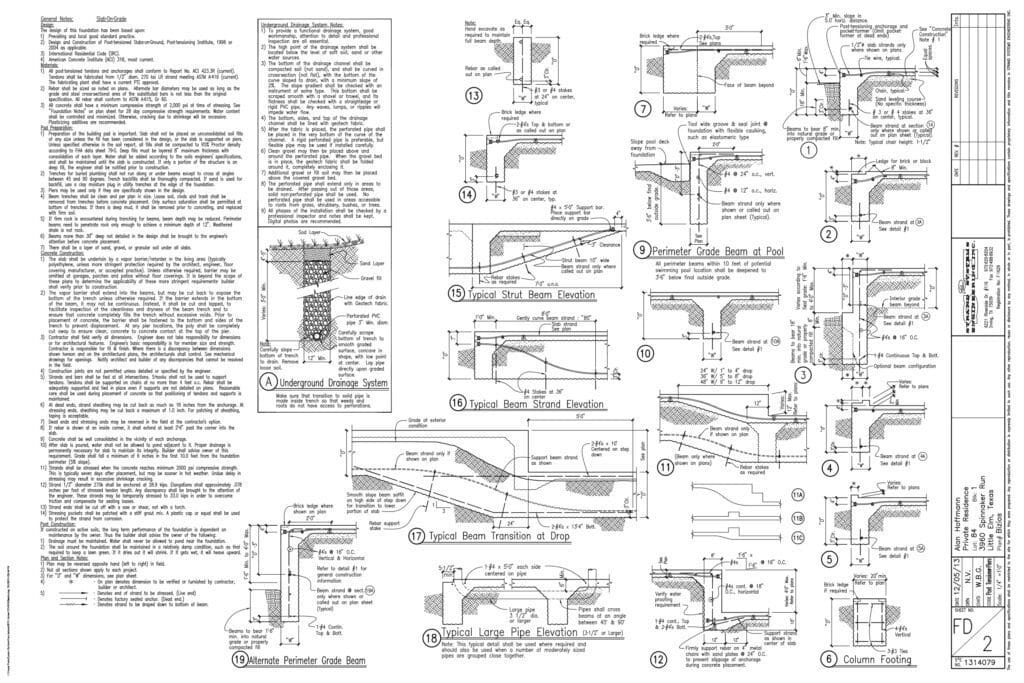Select Page

In Texas, there is no statute that defines failure for a residential foundation.  To the extent that definitions exist, they have been established by trade groups.

There are two groups that have produced written standards defining what constitutes a failure for a residential foundation.  The organizations are the Post Tensioning Institute, sometimes referred to as the PTI, and the American Society of Civil Engineers, also known as the ASCE.  Both standards are similar and use the same numerical criteria for determining what is a failure.  Both standards allow an evaluating engineer some discretion in determining, for a specific set of conditions, what constitutes a failure.  In addition to considering the numerical criteria, engineers may also consider cosmetic damage, functionality, and safety.  Many formal foundation warranties refer to or directly incorporate the standards published by the PTI and ASCE.

There are two numerical criteria that are used.  The first is for slope.  When a flat level surface is tilted, it is said to be sloping.  Slope is calculated as the ratio of vertical distance from high to low that occurs over a measured horizontal distance.  For example, if a foundation that measures 60 feet from side to side is tilted so that the high side is 4 inches above the low side, the ratio is 4 inches divided by 60 times 12 (converting the feet to inches) or 4/720.  A foundation is said to fail if the ratio exceeds .01 which is one percent.  How far out of level does a foundation that is 60 feet across have to be to fail?  The answer is 1% of 720 inches or 7.2 inches.

The second standard is for deflection.  The deflection calculation is a way of measuring curvature.  To measure deflection, you must have an elevation survey of a slab.  Generally, it is very helpful to convert the elevation survey to a contour diagram, which is in fact a topographic map of a foundation.  Once an elevation survey is prepared, cross-sections are prepared so that one can see how a foundation is shaped.  On a completed cross-section, a straight line is drawn from one end of a cross-section to the other end.  The maximum distance between the straight line and the surface of the foundation, shown on the cross-section, is measured in inches.  If the ratio computed by dividing the maximum distance from the straight line to the surface of the slab, by the distance from one end of the cross-section to the other exceeds 1/360, the slab fails the test.  For a slab that is 60 feet across, if the edges are at the same elevation, and the center is 1 inch lower than the edges, the ratio would be 1/720 or, after dividing the top and bottom by 2, .5/360.  .5/360 is less than 1/360, so the house would pass.  For our hypothetical 60 foot wide house, how far would the center have to be down for the slab to fail?  For a 60 foot wide home, the maximum allowable deflection is 2/720 (60 feet equals 720 inches).  To fail, the center of the house would need to be lower than the edges by at least two inches.

Shown below is a field elevation survey for a home.  Contour lines have been drawn in red and labeled.  The route of the cross-section runs from point “A” to point “B” and is shown on the diagram of the house as a wide green line.  Below the elevation survey, a plotted cross-section is shown, as is the deflection calculation, all in a graphic form.

Below the diagram of the house, is the plot of the cross-section.  On the plot of the cross-section, at each point where the cross-section line on the elevation survey passes over an elevation, the elevation is plotted.  For each elevation point, the pale green lines run from the elevation point to the plot of that elevation point on the cross-section.  By connecting the points plotted on the cross-section we actually draw the surface of the slab.  We can see the shape of the slab along the route of the cross-section.  In the example, for the house, we can see that the edges are high and the center is low.  We then measure the distance from line A-B, on the cross-section, to the surface of the slab, shown by the black line.The length of line A-B is 60 feet, which is 720 inches.  To convert 720 to 360, we divide 720 by 2.  Since we are dealing with a fraction, we have to divide the top, which is 2.3 inches, by two.  The resulting fraction is 1.15/360, which is greater than 1/360, so the house fails.

If you are filing a claim under a warranty, first check to see what standards are referenced.  If the PTI or ASCE are referenced, check to see if the reference includes only the numerical standards or if the references include each of the respective documents in their entirety.

If you think you need foundation repairs, call the professionals at Advanced Foundation Repair, LP or visit us at our website, foundationrepairs.com.

For those that are interested in reading the published standards, the document published by the PTI is Guide for Performance Evaluation of Slab-on-Ground Foundations PTI DC1-.8-18, and the document published by the ASCE is Guidelines for the Evaluation and Repair of Residential Foundations.